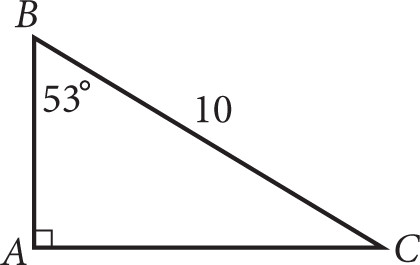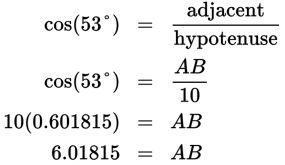# SAT Math Multiple Choice Question 461: Answer and Explanation

### Test Information

Question: 461

11.Based on the figure above, what is the approximate length of side AB?

• A. 6
• B. 7.2
• C. 8
• D. 8.5

Explanation:

A

Difficulty: Medium

Category: Additional Topics in Math / Trigonometry

Strategic Advice: ABC is a right triangle. You know the length of one side and the measure of one of the acute angles, which means you can use SOH CAH TOA.

Getting to the Answer: You know the length of the hypotenuse (10) and you're looking for the length of the side adjacent to (touching) the 53° angle, so use cosine. Set up a trigonometric ratio and solve for the length of AB. Make sure your calculator is set to degree mode.The length of AB is approximately 6.